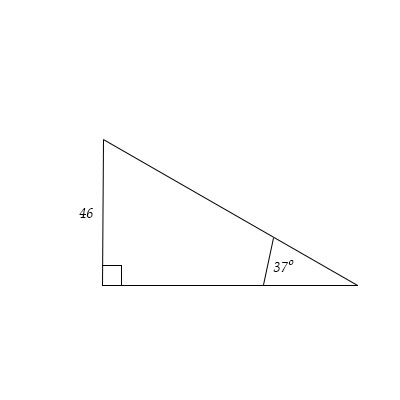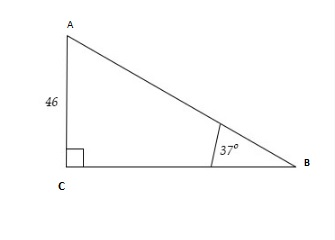# Solve the triangle:

## Question:

Solve the triangle:## Trigonometric Ratios:

The trigonometric ratios are used to find the length and the angle of the right angle and non-right angled triangles. The length sol found for each side of the triangle are termed as the solution of the given triangle

We will first mark the vertex of the triangle as shown in the figure below:Now we use the trigonometric ratios to get the other sides of the triangle:

{eq}\displaystyle \tan 37^{\circ \:}= \frac{46}{BC}\\ \displaystyle \Rightarrow BC=\frac{46}{\tan \left(37^{\circ \:}\right)}=61.04\\ {/eq}

Also we have:

{eq}\displaystyle \sin 37^{\circ \:}= \frac{46}{AB}\\ \displaystyle \Rightarrow AB=\frac{46}{\sin \left(37^{\circ \:}\right)}=76.4354\\ {/eq}

So this is the solution of the given triangle.